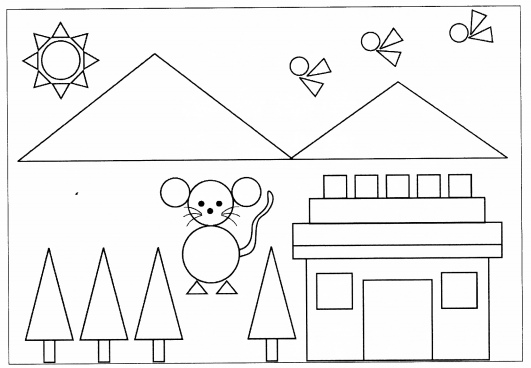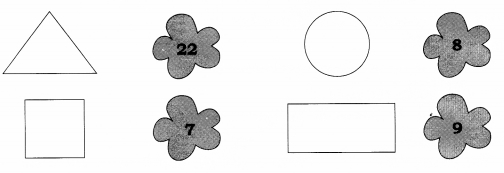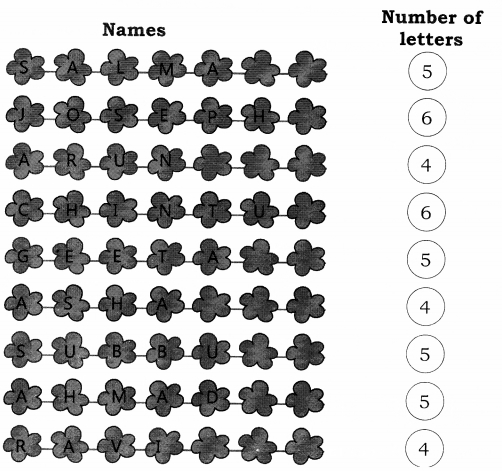NCERT Solutions for Class 1 Maths Chapter 9 Data Handling includes all the important topics with detailed explanation that aims to help students to understand the concepts better. Students who are preparing for their Class 1 exams must go through NCERT Solutions for Class 1 Maths Chapter 9 Data Handling. Going through the solutions provided on this page will help you to know how to approach and solve the problems.

Students can also find NCERT intext, exercises and back of chapter questions. Also working on Class 1 Mathematics Chapter 9 Data Handling NCERT Solutions will be most helpful to the students to solve their Homeworks and Assignments on time. Students can also download NCERT Solutions for Class 1 Maths Chapter 9 Data Handling PDF to access them even in offline mode.

Board: CBSE
Textbook: NCERT
Class: Class 1
Subject: Maths (Math Magic)
Chapter Name: Data Handling
Number of Questions – 3

## NCERT Solutions for Class 1 Maths Chapter 1 Shapes and Space

NCERT Solutions for Class 1 Maths Chapter 9 Data Handling are been solved by top teachers of LearnCBSE.in. All the solutions given in this page are solved based on CBSE Syllabus & NCERT guidelines. So before getting into the details of CBSE NCERT Solutions for Class 1 Math Magic Chapter 9 Data Handling let’s have an overview of list of topics and sub topics under this chapter.

• Count the Shapes in the given Figure
• Name the figure
• Count the number of letters in each name-word.

NCERT Textbook Page 109.Question 1.
Count the shapes in the above picture.Question 2.
Name the shapes
(a) that occurs the most
Triangle

(b) That occurs the least
Square

Question 3.
These children are friends.
(a) Count the number of letters in each name-word.(b) How many names have four letters?
Three

(c) How many names have five letters?
Four

(d) How many names have six letters?